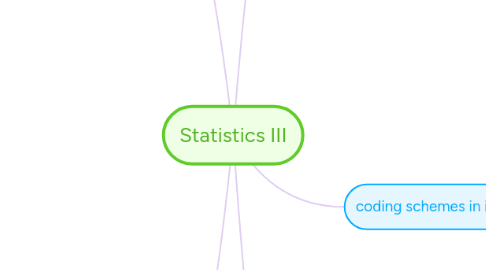# Statistics IIIL. Seeland
Laten we beginnen. Het is GratisStatistics III Door## 1. categorical/numerical IVs

### 1.2. different coding systems

1.2.1. dummy coding (reference coding)

1.2.2. unweighted effects coding

1.2.3. weighted effects coding

1.2.4. contrast coding

## 2. different coding schemes

### 2.1. effects coding

2.1.1. compare each group to the overall mean

2.1.2. B's=deviation from the overall mean

2.1.3. when all ni's are equal it doesn't matter which effects coding

2.1.4. unweighted effects coding

2.1.4.1. Mu=1/g ∑ Mi

2.1.4.2. used when

2.1.4.2.1. each group mean has the same weight

2.1.4.2.2. experimental design

2.1.4.2.3. differences in group means are not relevant

2.1.4.3. one group as base group (that you are least interested in) code as -1

2.1.5. weighted effects coding

2.1.5.1. Mw = (∑ ni*Mi)/(∑ ni)

2.1.5.2. used when

2.1.5.2.1. each group mean has a relative weight ni/∑ni

2.1.5.2.2. sampling proportion reflects population proportions

2.1.5.3. one group as base group (that you are least interested in) code as -(ni/nbasegroup)

2.1.6. model Y=B0+B1C1+B2C2+B3C3

### 2.2. dummy coding

2.2.1. assign reference group

2.2.2. model Y=B0+B1C1+B2C2+B3C3

### 2.3. contrast coding

2.3.1. when there is a specific hypothesis that can be used for contrasts

2.3.2. rules

2.3.2.1. for each Ci: ∑Chi=0 h=groups

2.3.2.2. for each pair of variables: ∑Chi*Chj=0

2.3.2.3. for each variable the difference between largest and smallest value must be 1

2.3.3. model Y=B0+B1C1+B2C2+B3C3

## 3. coding schemes in interaction with other IV's

### 3.1. 2x2 interaction

3.1.1. model: Y=B0+B1C(D)+B2C(E)+B3C(D*E)

3.1.2. for all coding systems R², F-test and t-test (of the interaction) are the same

3.1.3. for contrast coding and unweighted effects coding all t-tests are the same

3.1.4. interpretation of the B's changes with coding scheme

### 3.2. ga by gb interaction

3.2.1. categorical IV's with >=2 categories each

3.2.2. two steps

3.2.2.1. which main effects and interactions should be indluded in the model?

3.2.2.1.1. use F-test to test for significance

3.2.2.1.2. four different types of sums of squares (SS)

3.2.2.1.3. when you include the interaction always include the original variables and every lower-order interaction

3.2.2.2. chose adequate coding, fit the model and interpret

3.2.3. model: Y=B0+B1C(D)+B2C(E)+B3C(D*E)

### 3.3. nominal by continuous interaction

3.3.1. two steps

3.3.1.1. which main effects and interactions should be indluded in the model?

3.3.1.1.1. use F-test to test for significance

3.3.1.2. chose adequate coding, fit the model and interpret

3.3.1.2.1. interpretation of B's: always adjusted for other variables being in the model

3.3.2. model: Y=B0+B1C1+B2C2+B3x2+B4C1x2+B5C2x2

## 5. repeated measurement (M)ANOVA

### 5.1. univariate

5.1.1. ANOVA: SSt=SSb+SSw RM-(M)ANOVA: SSt=SSb+(SSb1+SSe)

5.1.2. SSb1 = random factor

5.1.3. removes individual differences between subjects (SSb1) from error term (SSw)

### 5.2. multivariate

5.2.1. each measurement is a DV

5.2.2. different multivariate tests to test if measurements differ from each other

5.2.2.1. use Wilks' Λ (lambda) as default

### 5.3. assumptions

5.3.1. independence of observations

5.3.2. normality of each subgroup

5.3.3. compound symmetry (only univariate)

5.3.3.1. homogeneous variances and covariances across treatment levels/time points

5.3.3.2. not realistic in practice -> use sphericity: all variances of pairwise comparisons are equal

5.3.3.2.1. Mauchly's test

### 5.4. which one to use?

5.4.1. multivariate

5.4.1.1. if sphericity is violated (ɛ < .7) and n>k+10

5.4.2. univariate

5.4.2.1. if sphericity holds (ɛ>.7)

5.4.2.2. if sample sizes are small

### 5.5. decomposing the within-factor effect

5.5.1. using polynomial contrasts (trend analysis)

5.5.2. using post-hoc procedures

5.5.2.1. if ɛ>.7 use Tukey

5.5.2.2. if ɛ<.7 use Bonferroni